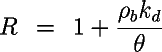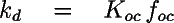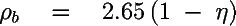# EPA On-line Tools for Site Assessment Calculation

 11 of 67

### Retardation Coefficient

The retardation coefficient expresses how much slower a contaminant moves than does the water itself.where R is the retardation coefficient, rb is the bulk density, kd is the sorption coefficient, and q is the porosity.

#### Background

A simplified method of accounting for sorption is in common usage. This method is based on the assumption that sorption of organics occurs to the naturally occurring organic carbon in the aquifer. Obviously, the properties of the chemical are important also. Thus a sorption coefficient, kd (also called the soil/water distribution coefficient) is defined from the fraction organic carbon, foc in the aquifer and the organic carbon partition coefficient, Koc of the chemical:The Koc values used in OnSite are tabulated on the chemical properties page. Use of Koc --- foc as input parameters refects hydrophobic sorption theory and the observation that sorption of organic chemicals occurs as a partitioning to aquifer organic carbon. These two parameters are used internally to calculate the solid/water distribution coefficient, kd

#### Bulk Density

The bulk density appears in the definition of the retardation coefficient. Bulk density appears because concentrations in the water phase are measured relative to volume of water and sorbed concentrations are measured relative to the solid mass.where the factor of 2.65 is the density of quartz sand, giving the bulk density units of g/cm3.

 Previous Top ^ Next

Home | Glossary | Notation | Links | References | Calculators

Contact Jim Weaver to ask a technical question on this material.